# DeepSig’s 2018 Data Set: 2018.01.OSC.0001_1024x2M.h5.tar.gz

The third DeepSig data set I’ve examined. It’s better!

Update February 2021. I added material relating to the DeepSig-claimed variation of the roll-off parameter in a square-root raised-cosine pulse-shaping function. It does not appear that the roll-off was actually varied as stated in Table I of [R137].

DeepSig’s data sets are popular in the machine-learning modulation-recognition community, and in that community there are many claims that the deep neural networks are vastly outperforming any expertly hand-crafted tired old conventional method you care to name (none are usually named though). So I’ve been looking under the hood at these data sets to see what the machine learners think of as high-quality inputs that lead to disruptive upending of the sclerotic mod-rec establishment. In previous posts, I’ve looked at two of the most popular DeepSig data sets from 2016 (here and here). In this post, we’ll look at one more and I will then try to get back to the CSP posts.

Let’s take a look at one more DeepSig data set: 2018.01.OSC.0001_1024x2M.h5.tar.gz.

The data set is from 2018 and is associated with the O’Shea paper The Literature [R137]. It, like the other two I’ve analyzed (here and here) [Update: A fourth.], is currently available on the DeepSig website. The data set contains 24 different signals each of which is provided at each of 26 different SNR values. For each signal and SNR combination, there are 4096 instances, and each instance always has length 1024 samples. The data are stored in an HDF5 format, so I used normal HDF5 tools to extract the signals, such as h5dump. I’ll show you below how I learned all these attributes of the data set.

When you unzip and untar the archive, you get three files: LICENSE.TXT, classes.txt, and GOLD_XYZ_OSC.0001_1024.hdf5. The latter is where the data is, and classes.txt looks like this:Figure 1. The classes.txt file included in the zipped archive. It is consistent with the DeepSig website description that says the archive contains 24 different signal types.

The data itself is contained in the GOLD*hdf5 file, which requires a bit of examination to understand, and to attempt to connect to the classes shown in classes.txt.

I used hdf5-file tools available under linux (Ubuntu and Fedora Core in my case) to discover the structure of the data. The first step is to use h5dump to query the file about the data sets it contains. This leads to the following terse output:

So far from the description on DeepSig’s website, and the data description in the associated paper (The Literature [R137]), we know that there are 24 signals, the intended SNR range is$[-20, +30]$ dB, and that each individual signal data-record will always be 1024 samples in length, presumably complex-valued samples.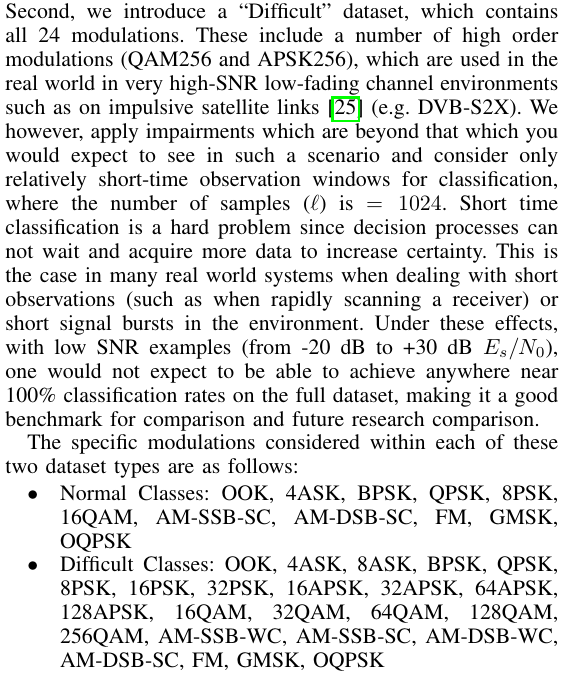Figure 3. Extract from The Literature [R137] describing the data set under consideration in this post.

But we don’t know exactly how many of each signal there are nor the signal parameters, such as symbol rate. And, crucially, we don’t yet know which signal type is associated with each data record. So let’s look at the three datasets /X, /Y, and /Z.

### The Datasets /X, /Y, and /Z

When we attempt to dump the contents of dataset /X, we get an output with the following header:

The DATASPACE SIMPLE line indicates that the dataset /X is three-dimensional, having 2555904 records in the first dimension, 1024 in the second, and 2 in the third. So we can guess that there are 2555904 total signals, each has 1024 samples, and those samples are complex, so need two values per sample. That explains the three dimensions.

Looking at dataset /Y, we see

So the /Y dataset is two-dimensional, with 2555904 records in the first dimension and 24 in the second. As we can see from the first few records, the 24-element vector for each record is a binary vector with only one value equal to 1 and the rest equal to 0. So this must be the modulation-type indicator. Looking good! Mysterious, and requiring some sleuthing, but good.

Turning to dataset /Z, we see

So /Z is a single vector of length 2555904 and with starting values of -20. If you look at the bottom of the vector, the value is 30, so this is the SNR-parameter dataset. There are 26 distinct SNR parameters, ranging from -20 to +30 in steps of 2. The parameter is held constant for 4096 values, then moves on to the next value. Once it gets to +30, the next value is again -20. The period of this parameter is therefore 4096*26 = 106496. To make this concrete, here are some plots of the SNR parameter:

So it looks like the SNR parameter is held constant for 4096 data records, and the SNR parameter sequence repeats after 4096*26 = 106496 data records, indicating that the signal class is likely held constant for 106496 data records. Turning back to the signal-class indicator dataset /Y, we see that the binary indicator vector switches from a one in the first location to a one in the second location exactly after 106496 data records:Figure 10. From dataset /Y, the signal-class indicator dataset, we see that the 106497th record is the first one corresponding to the signal class with a ‘1’ in the second location (the second signal class). Note that the read-out of the data records uses numbering starting with zero.

The signal-class indicator dataset /Y isn’t free of mysteries, but almost. There are a few places where the indicator as output by h5dump doesn’t quite make sense: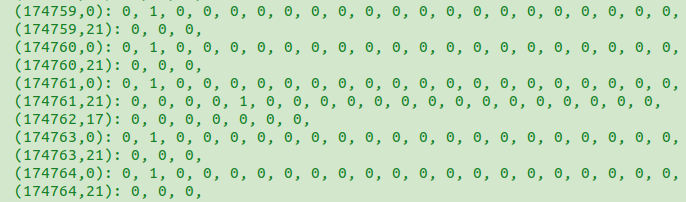Figure 11. The signal-class indicator vector in dataset /Y does not appear to be correct for records 174761 and 174762, or at least the formatted output of the indicator vector is flawed (the output of h5dump).

Presumably the signal-class indicator vector position is a map into the classes.txt set of strings I showed at the top of this post. So if the vector shows a 1 in the first position, then that data record would correspond to the 32PSK signal type. How could we verify that?

### Analysis of Extracted Data Records

Let’s try to analyze some of the data records we can extract from the hdf5 file and see if they have characteristics that match the corresponding signal type as determined by the associated position of the 1 in the signal-class vector of dataset /Y.

The main analysis is for a subset of data records for each signal type. I used h5dump to extract one example of each SNR condition for each signal type. Since I don’t really know the signal types in classes.txt conform to the signal-class indicator vector in the archive, I’m just going to refer to each type in terms of the offset into the hdf5 file. We know that each new signal type starts at a data-record offset of$k*106496$, so I’ll refer to the signals in terms of the offset$k$. I use h5dump commands like this:

h5dump -d /X -k 1,1,1 -s $offset,0,0 -S$stride,1,1 -c \$num_blocks,1024,2 GOLD_XYZ_OSC.0001_1024.hdf5

where offset is$k$, stride is 4096, and num_blocks is 26. I do this for all 24 offsets (starting with 0). This produces 24 data records each with length 26*1024 = 26624 complex samples.

First let’s look at the modulus of the 24 signals:Figure 12. The modulus (magnitude) of each of the extracted signals. There is one 1024-sample subblock of data for each of the 26 distinct SNR parameters for each signal. SNR increases as the x-axis value increases.

Since the SNR parameter increases to the right, but the moduli decrease (generally), the SNRs are achieved by decreasing the noise power, thereby decreasing the total power.

The closest thing to an exactly constant-modulus signal is Offset 21, but Offset 22 is also close to constant compared to the other signals. Offsets 17 and 18 are strange and non-monotonic in the modulus behavior. But not much else is evident. Let’s turn to plots of the real and imaginary parts:Figure 13. The real part of the 24 signals. Here we are only plotting the final subblock, which corresponds to the SNR parameter of +30.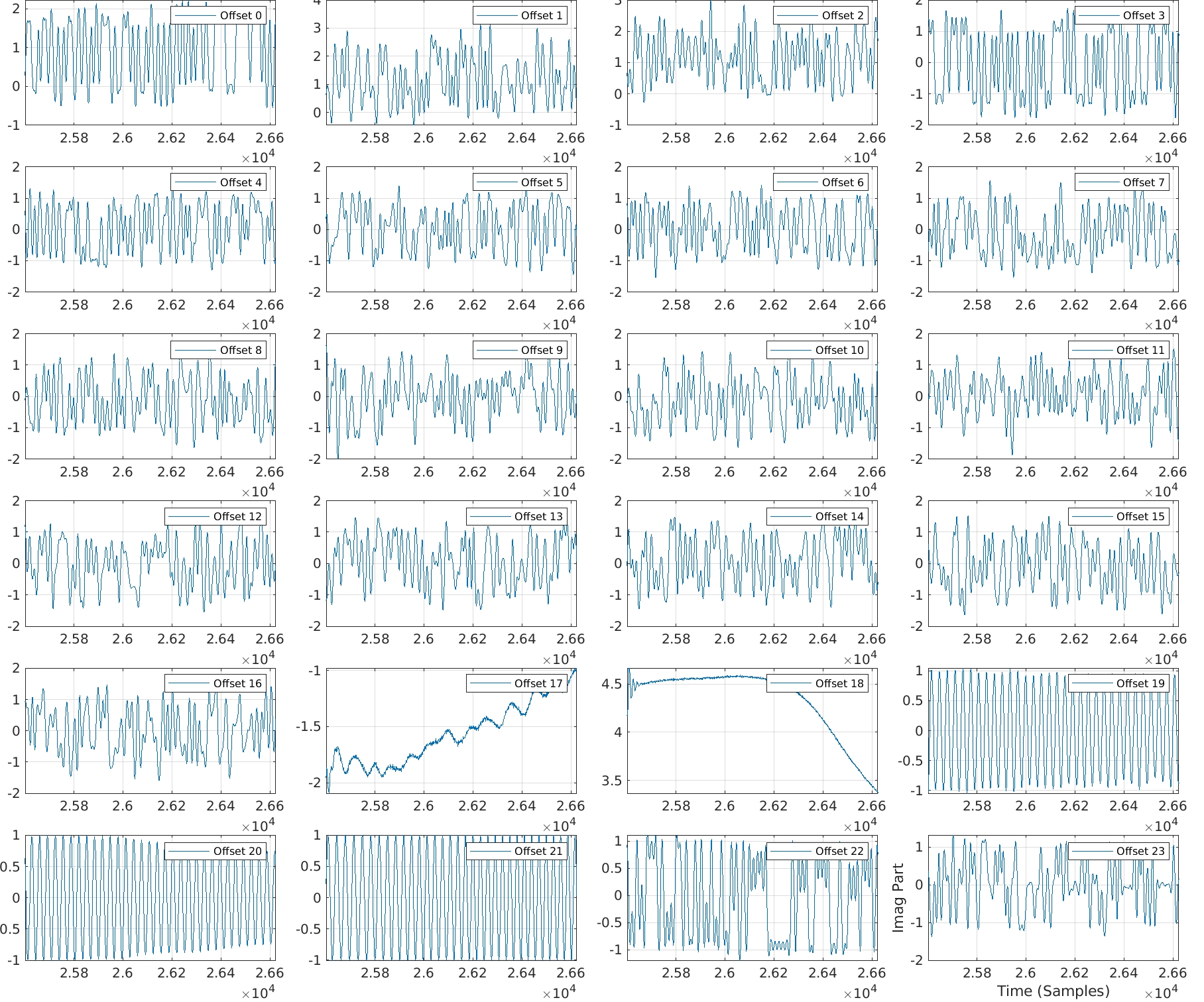Figure 14. The imaginary part of the 24 signals. Here we are only plotting the final subblock of each signal, which corresponds to the SNR parameter of +30.

From the real and imaginary plots, we see that most of the Offsets produce approximately zero-mean sequences, but Offsets 0-2, 17, and 18 do not. Finally, let’s look at some power spectra. First I’ll show the PSDs for each signal, taking into account all 26624 samples and using the TSM for spectrum estimation: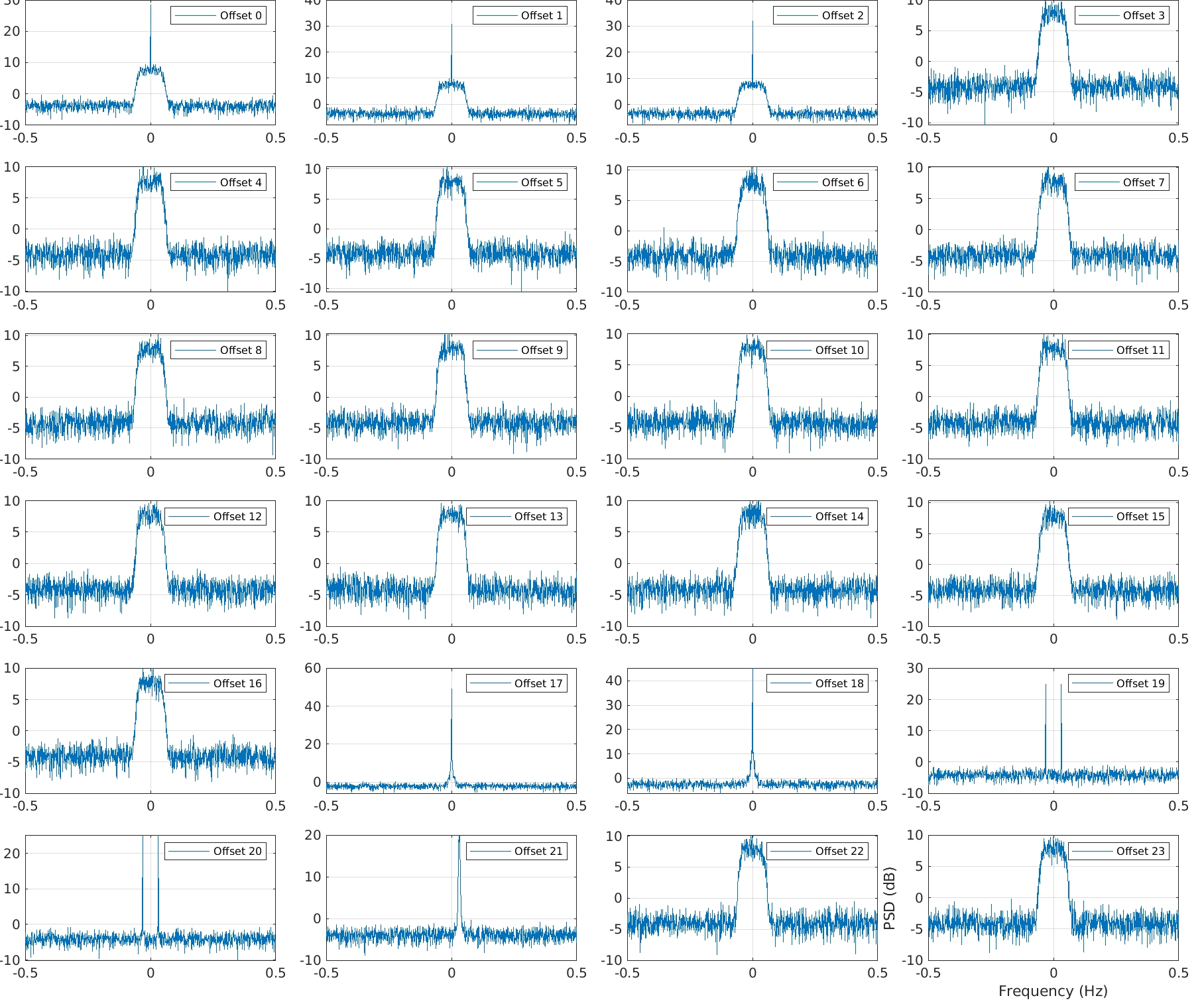Figure 15. Power spectral density estimates for the 24 extracted signals. Recall here we are processing extracted signals that consist of one 1024-point subblock per unique SNR value per signal type (Offset).

The first three Offsets (0, 1, and 2) produce a signal with a QAM/PSK-like spectral shape and also an additive sine-wave component, like what you see for OOK signals. These are followed by 14 PSDs that look like garden-variety PSK/QAM PSDs for a signal with symbol rate of around 1/10. Those are followed by Offsets 17-21, which show signal types that are very nearly sinusoidal (Offsets 17, 18, 21) or periodic (Offsets 19 and 20). Finally, there are two more garden-variety PSK/QAM PSD shapes. Since these PSDs take into account the full range of SNRs (-20 to +30), I’ll show the PSD estimates just for the final subblock (SNR parameter of +30) to get a low-noise look at each signal: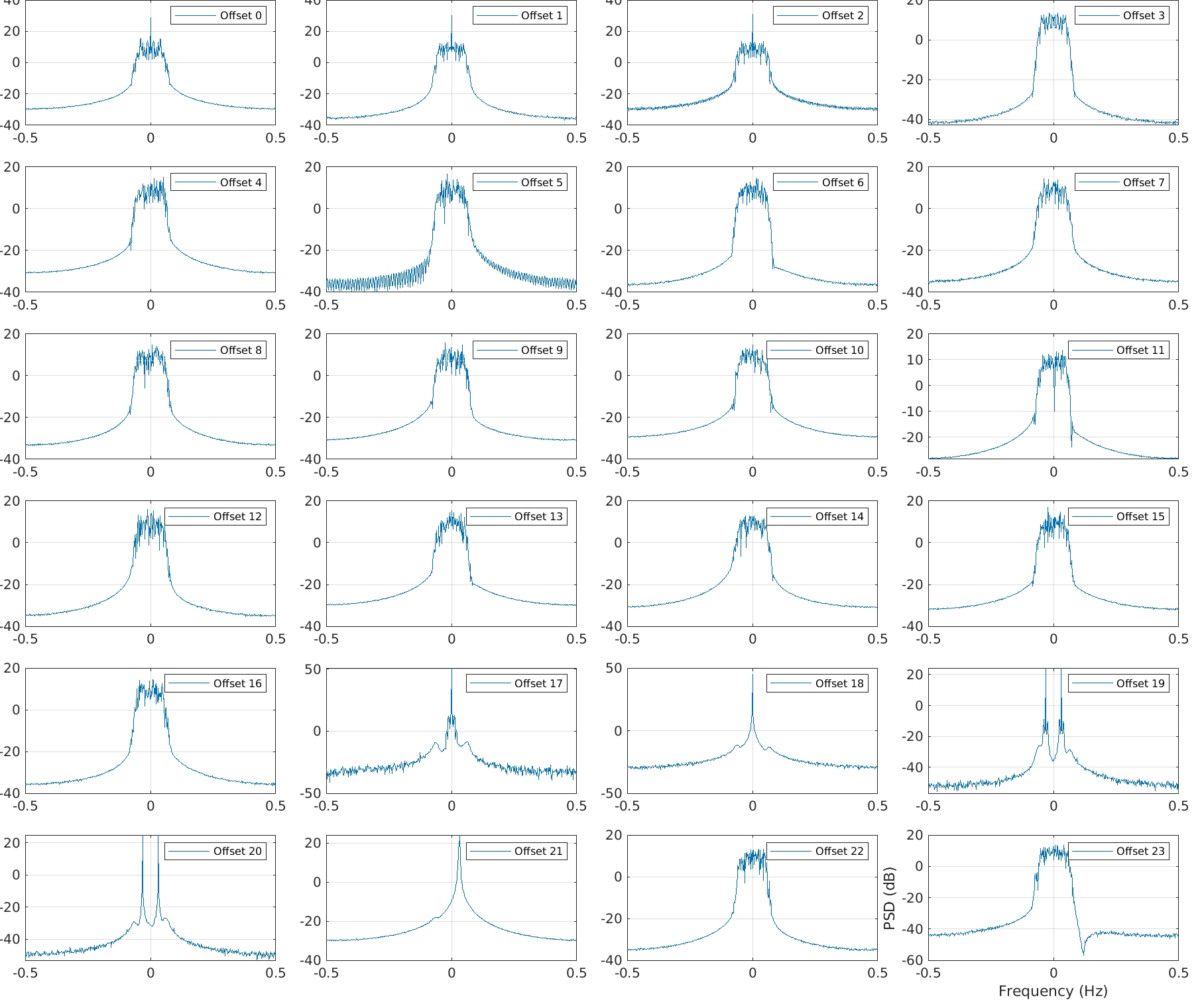Figure 16. PSD estimates for a single 1024-point extracted data block for each signal and the largest SNR parameter value of +30.

If the mapping between the signal-class strings in classes.txt matched this data, we would expect to see an approximately constant modulus (applied channel effects can ruin the constancy of the modulus) for FM, which would be Offset 3. But the closest to constant modulus is Offset 21. Offset 22 is the next closest. So the mapping is in serious doubt.

If we return to The Literature [R137], and examine the proffered confusion matrices there, we see a different ordering of the signal classes:

This ordering is more consistent with the signal-class indicator vector in dataset /Y. The first signal is OOK, which should have a PSD with a typical QAM/PSK bump and an impulse midband, which it does in Figure 15. The final two signals in the confusion matrix are GMSK and OQPSK, which also have PSK/QAM PSDs and should not have impulses, and that is what we see in the final two PSDs (Offsets 22 and 23) in Figure 15.

Between 8ASK and AM-SSB-WC, there are 14 garden-variety types in the confusion matrix, which is consistent with Figure 15. Finally, the analog signals correspond to Offsets 17-21 in the confusion matrix and those Offsets correspond to the PSDs in Figure 15 that are the most non-PSK/QAM in appearance.

So the mapping provided by DeepSig in classes.txt is incorrect, but a correct one is possibly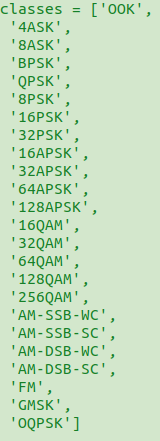Figure 18. Possible corrected classes for the DeepSig 2018 data set. This is the hypothesized order of appearance of the signals in the dataset /X.

To check that the rather severe subsampling of the data records for each modulation offset above didn’t miss anything significant, I extracted every tenth 1024-point subblock from the archive for Offset = 3. Here are the PSDs:

### Square-Root Raised-Cosine Roll-Offs in the Data Set

In [R137] we find the table of random variables that are applied to the signals in Table I:

In Table I,$\alpha$ is the variable the represents the roll-off parameter (don’t confuse it with cycle frequency!), so that the data set should exhibit signals with excess bandwidths in the range of$10-40$%. To check this I would like to pass examples through a modulation-recognizer that is sensitive to pulse-shaping functions, but the authors’ preoccupation with very short data records inhibits this approach. Let’s see how far we can get by looking at some PSDs.

I estimated the PSDs for the$4096$ strongest Offset-3 signals and plotted them on a single set of axes as shown in Figure 19. Overlaid on those$4096$ PSDs are three additional PSDs corresponding to PSK signals with roll-offs$\alpha$ of$0.1, 0.2,$ and$0.4$. (All estimates use identical methods and spectral resolution parameters.) I have to conclude that either the range of roll-offs is much smaller than claimed or the pulse-shaping function used by DeepSig is not the usual square-root raised-cosine pulse function. To plot the three colored PSD lines in Figure 19 requires that I chose a symbol rate. My best guess is exactly$1/8 = 0.125$ because when I pass the$1024$-point blocks through a blind non-conjugate cycle-frequency estimator (such as the FAM or SSCA), the only consistently detected value is$0.125$.Figure 19. Estimated PSDs for each of blocks of the signal at Offset 3, along with CSP-Blog generated PSDs for PSK signals with several square-root raised-cosine pulse-shaping filter roll-offs. The latter signals employed a symbol rate of and carrier offset of zero.

### Discussion

The impulses in 4ASK and 8ASK (Offsets 1 and 2) are expected because the constellations were probably all non-negative numbers along the real-axis, which is a typical definition of ASK, so that checks.

The five analog waveforms don’t make sense to me. They are essentially impulsive in the frequency domain, and there doesn’t appear to be much difference between those “with carrier” and those with “suppressed carrier.” In particular, suppressed-carrier signals should not contain impulses in their spectra. It looks like the AM and FM signals are being driven by a sinusoidal message signal (non-random).

Otherwise, this is a much better data set than the other two DeepSig data sets I’ve analyzed (see All BPSK Signals and More on DeepSig’s RML Data Sets).

It still suffers from the “one BPSK signal” flaw, because it looks like the symbol rate and carrier offset never significantly change (see Table I in The Literature [R137]), and I have come to the conclusion that the excess bandwidth (roll-off parameter$\alpha$ for the square-root raised-cosine pulse-shaping filter) is not actually varied. (Compare this to my Challenge Data Set.)

The data set also suffers from the preoccupation with very short data records. This prevents verification and other kinds of analysis and comparison. If the data records were made longer, presumably a machine learner could still train and test using a subset of each data record (use the first$N$ samples of each data record with length$M \gg N$), so there isn’t any disadvantage in making longer records except the size of the archive increases.

As usual, let me know if I’ve erred or if you have a relevant comment in the Comments section below.I'm a signal processing researcher specializing in cyclostationary signal processing (CSP) for communication signals. I hope to use this blog to help others with their cyclo-projects and to learn more about how CSP is being used and extended worldwide.

Posted on

## 12 thoughts on “DeepSig’s 2018 Data Set: 2018.01.OSC.0001_1024x2M.h5.tar.gz”

1.Peter says:

thanks for that interesting analyses of the radio ML datasets. Assuming these are correct: This would be a serious issue to the scientific community working in that topic! Have you received any statement or comment from Tim O’Shea?

1.Chad Spooner says:

Thanks for stopping by and leaving a comment Peter! Welcome.

The first post I did on Machine Learning for Modulation Recognition was a critical review of the RML paper by O’Shea, Clancy, and Corgan. That was in early 2017. Tim left this comment, and you can read my reply to that comment, which was never answered. Subsequently, I met Tim and tried to work on some ML stuff with him. I know for a fact he has my Data Set for the Machine Learner’s Challenge. But he never disclosed to me his results.

Since then, he hasn’t commented on any of the data-set analysis posts here, here, and here. This is all fine with me.

1.Fatih ÇORUK says:

Hi Mr. Spooner. I am Electrical&Electronics Engineering 4th grade student in Ankara University. My project homework is about over the air deep learning based radio signal classification. And i see that you have worked on this paper and written its codes. Could you please share the python codes? If you answer me positively, then i will be thankful to you.

1.Chad Spooner says:

Fatih: I’m sorry to tell you that you are mistaken. I did not work on the paper associated with this data set ([R137] in The Literature). I did analyze the data set for the benefit of the modulation-recognition community. I suppose you could try contacting the authors of [R137].

1.Fatih ÇORUK says:

I see, thank you sir.

2.Mutescu Marian says:

Hello Mr. Spooner!
I am currently pursuing my Phd. on the topic of Spectrum Sensing and I found valuable information in your work. Your analysis on the DeepSig dataset is the most detailed I’ve found so far. I have noticed that in this article you state that each modulation class is maintained for 106496 samples in the /Y dataset ( also the SNR is ranged from -20 to +30 in the /Z dataset for 106496 samples ) , but each signal you extracted from the /X dataset has only 26624 samples. Are there multiple instances of the same signal for each SNR (4096 instances), from which you extracted only one instance or am I missing something? Please clarify this aspect for me. Also I am looking forward to tackle the dataset you provided in the “Data Set for the Machine-Learning Challenge” article. Thank you!

1.Chad Spooner says:

Welcome to the CSP Blog Mutescu (or is it Marian?)!

I will answer your question. But first, I need you to answer one of mine. You say

Your analysis on the DeepSig dataset is the most detailed I’ve found so far.

I have seen zero other analyses of the DeepSig data sets. I’ve seen only applications of ML to the data set.
Can you please write down here links to or citations to the other analyses that you’ve seen?

1.Mutescu Marian says:

You are right, there are zero official analyses on this dataset. The other “analyses” I mentioned were a couple of answers and opinions scrambled trought forums.

2.Chad Spooner says:

I think you’re asking about Figure 12. I extract one example of each modulation type (what I’m calling the offset in the post) for each SNR value. I then concatentate those data records. So for a particular signal type (offset), I have 26 (there are 26 SNRs) complex vectors with length 1024. Concatenating these 26 vectors leads to a complex vector with length 26*1024 = 26624. I then plot the magnitude (modulus) of that vector on one of the axes in Figure 12. So each little subplot in Figure 12 shows 26 different versions of a single signal type, just with different SNRs.

Are there multiple instances of the same signal for each SNR (4096 instances), from which you extracted only one instance or am I missing something?

Yes, there are multiple instances of the same signal for each SNR, but I only extracted one instance of each SNR for each signal to create the 24 subplots in Figure 12.

For the video of power spectrum estimates near the end of the post, I extract lots of instances of each SNR but for only one signal type (offset 3).

Does that clarify the post for you? Let me know…

1.Mutescu Marian says:

3.Laura says:
1.Chad Spooner says: